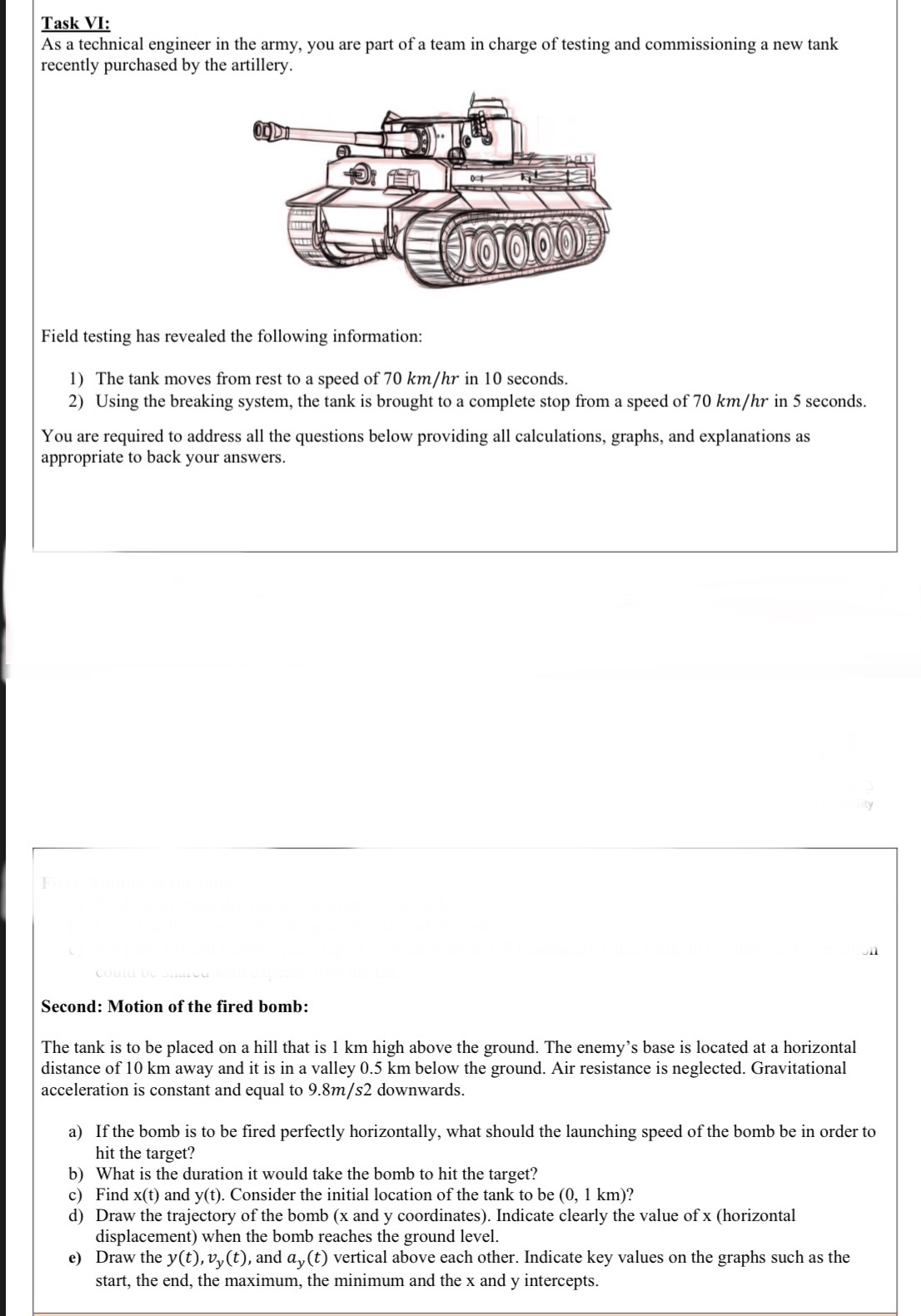# The tank is to be placed on a hill that is 1 km high above the ground. The enemy's base is located at a horizontal distance of 10 km away and it is in a valley 0.5 km below the ground. Air resistance is neglected. Gravitational acceleration is constant and equal to 9.8m/s2 downwards. a) If the bomb is to be fired perfectly horizontally, what should the launching speed of the bomb be in order to hit the target? b) What is the duration it would take the bomb to hit the target? c) Find x(t) and y(t). Consider the initial location of the tank to be (0, 1 km)? d) Draw the trajectory of the bomb (x and y coordinates). Indicate clearly the value of x (horizontal displacement) when the bomb reaches the ground level. e) Draw the y(t), v,(t), and a, (t) vertical above each other. Indicate key values on the graphs such as the start, the end, the maximum, the minimum and the x and y intercepts.

Question

I need someone to solve physics questions is necessaryhelp_outlineImage TranscriptioncloseThe tank is to be placed on a hill that is 1 km high above the ground. The enemy's base is located at a horizontal distance of 10 km away and it is in a valley 0.5 km below the ground. Air resistance is neglected. Gravitational acceleration is constant and equal to 9.8m/s2 downwards. a) If the bomb is to be fired perfectly horizontally, what should the launching speed of the bomb be in order to hit the target? b) What is the duration it would take the bomb to hit the target? c) Find x(t) and y(t). Consider the initial location of the tank to be (0, 1 km)? d) Draw the trajectory of the bomb (x and y coordinates). Indicate clearly the value of x (horizontal displacement) when the bomb reaches the ground level. e) Draw the y(t), v,(t), and a, (t) vertical above each other. Indicate key values on the graphs such as the start, the end, the maximum, the minimum and the x and y intercepts. fullscreen

### Want to see this answer and more?

Experts are waiting 24/7 to provide step-by-step solutions in as fast as 30 minutes!*

*Response times may vary by subject and question complexity. Median response time is 34 minutes for paid subscribers and may be longer for promotional offers.
Tagged in
Science
Physics

### Kinematics